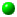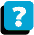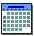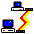Module 4.3 Notes "Comparing Two Samples Using a Nonparametric Test"

 Index to Module 4 Notes4.1 Comparing 2 Independent Samples of Numerical Data4.2 Comparing 2 Related Samples of Numerical Data4.3 Comparing 2 Samples Using a Nonparametric Test4.4 Comparing Multiple Samples: One Factor4.5 Comparing Multiple Samples: Two Factors4.6 Comparing Multiple Samples: A Nonparametric Test

In Module Notes 4.1, we indicated that there are two assumptions when doing statistical tests involving the comparison of two samples to make an inference about population means. The first is that the samples are drawn from normally distributed populations (or samples are large without extreme skew); and the second is that the samples have equal variances. When these assumptions are met, we use the t-Test for Two Independent Samples Assuming Equal Variances. In the situation where the variances are not equal, but the parent populations are normally distributed (or samples are large without extreme skew) , we use the t-Test for Two independent Samples Assuming Unequal Variances.

What if we cannot make the assumption that the parent populations from which the samples were drawn are normal, especially when we are working with small samples? Then a safe alternative is to use a nonparametric test, rather than the parametric tests we have been using. Parametric tests are those tests such as the t-Test and the F-Test, which rely upon statistical distributions and assumptions about the distributions for analysis about population parameters. Nonparametric tests do not rely upon statistical distributions. Rather, nonparametric tests for numerical data, such as the one discussed in this module, make inferences about populations by studying sample measures of location, such as the median. Rather than using all of the information available in samples, these nonparametric tests typically use only the ranks of the observations (Aczel, p. 625). As such, these type of tests work well with ordinal as well as interval or ratio scale numerical data.

This set of Module notes introduces a nonparametric test for the study of two independent samples called the Wilcoxon Rank Sum Test (also goes by Mann Whitney U Test, Aczel, p. 644). This will be followed by a nonparametric test for the study of two related (dependent) samples called the Wilcoxon Signed-Rank Test.

Wilcoxon Rank-Sum Test for Comparing Two Independent Samples

I will continue with the Brand of Gas data. I am interested in comparing cars tested with Brand A against those tested with Brand B. The only assumptions required for the Wilcoxon Rank Sum Test are that the samples are random, and that observations drawn for each sample are independent from each other. Therefore, this test could not be used for the paired sample situation of Module 4.2.

The general hypotheses for the Wilcoxon Rank-Sum Test are (Aczel, p. 644):

H0: The distributions of the two populations are identical
Ha: The distributions of the two populations are not identical

If we want to state the hypotheses in terms of population medians (Levine, 1999), then we are stating that if there is a difference in the populations, it is a difference in location (Aczel, p. 645):

H0: MedianA = Median B
Ha: MedianA =/= MedianB

Worksheet 4.3.1 provides the original data. Note I added two columns and ranked the data within each group.

Worksheet 4.3.1

 Brand A Brand B Brand A Brand B 20 20 14 18 20 20.5 15 18.5 19 18.5 16 19 16 20 17 19 15 19 19 20 17 19 20 20 14 18 20 20.5

To perform the Wilcoxon Rank-Sum Test, we replace the observations in the two samples of size n1 and n2 with their combined ranks. There will be n = n1 + n2 ranks. We can let Brand A be group 1 and Brand B be group 2. So, for our example:

Eq. 4.3.1: n = n1 + n2 = nA + nB = 7 + 7 = 14 ranks

We assign the ranks so that rank 1 is given to the smallest of the combined number, rank 2 to the second-smallest, and so forth. If several values are tied, a common practice is to assign each tied observation "the average of the ranks that would otherwise have been assigned had there been no ties. "

A simple way of assigning the ranks in Excel is shown in Worksheet 4.3.2.

Worksheet 4.3.2
 Brand A Brand B A Ranks B Ranks 14 1 15 2 16 3 17 4 18 5 18.5 6 19 8 19 8 19 8 20 11.5 20 11.5 20 11.5 20 11.5 20.5 14 T1 = 41 T2 = 64

The smallest number is 14, so it gets rank 1, followed by 15, 16, 17, 18 and 18.5 which get ranks 2, 3, 4, 5, and 6 respectively. The next three numbers are 19, 19 and 19 - which are ties. Since these are to get the ranks 7, 8 and 9, we average the ranks and give each of the 19's the rank of 8. We then proceed through the data until we finish by assigning rank 14 to the number 21. Note how I maintained group identity.

The Wilcoxon Rank-Sum Test Statistic is T1, where T1 is assigned to the group with the smallest sample size. If the groups have the same sample size, as in our example, the assignment is arbitrary. I'll assign T1 to the first group, Brand A. T1 is the sum of the ranks in that group, as shown in Worksheet 4.3.2. Note that T2 is the sum of the ranks in the second group. Equation 4.3.2 shows a check on the rankings:

Eq. 4.3.2: T1 + T2 = n * (n + 1) / 2= 14 * (14 + 1) / 2 = 105

Since T1 + T2 equals 105 (41 + 64), our rankings check.

The Wilcoxon Rank Sum Test Statistic available in Excel is the Z for samples over size 10 (we are a little shy of that with samples of size 7, but I wanted to use a small size problem to illustrate the technique). To compute the Z for this test, we need the Wilcoxon Rank Sum formula for the mean and the standard deviation of T1.

Eq. 4.3.3: MeanT1 = n1(n+1)/2 = 7(14+1)/2 = 52.5
Eq. 4.3.4: Std DevT1 = Sq Rt { [ (n1n2(n+1) ] / 12 } =
Sq Rt { [7*7(14+1)] 12 } = 7.826

Eq. 4.3.5: Z =(T1 - MeanT1) / Std DevT1 = (41-52.5) / 7.826 = -1.47

We can use the Excel Function =NORMSDIST(-1.47) to get the p-value. The p-value returned by =NORMSDIST(-1.46) in an Excel Cell is 0.07, which represents the cumulative probability of a z-score less than or equal to -1.47. Since two times this p-value (2 * 0.07 = 0.14) is greater than 0.05, we fail to reject the null hypothesis and conclude the populations, from a location point of view, are the same (no difference in the medians). This is similar to our conclusion from the t-Test for Two Samples in Module Notes 4.1. Note that I doubled the p-value because the alternative hypothesis is two-tailed.

Let's do one more example, this time comparing Brand A against Brand C. Worksheet 4.3.3 provides the data.

Worksheet 4.3.3
 Brand A Brand C Brand A Brand C 20 20 14 20 20 26 15 23 19 23 16 23 16 24 17 23 15 23 19 24 17 25 20 25 14 23 20 26

Worksheet 4.3.4 provides the rankings.

Worksheet 4.3.4
 Brand A Brand C A Ranks C Ranks 14 1 15 2 16 3 17 4 19 5 20 7 20 7 20 7 23 10 23 10 23 10 24 12 25 13 26 14 Sum of Ranks = T1 = 29 T2 = 76

Equations 4.3.1 - 4.3.4 are identical to the first example since we have the same sample sizes in the two groups. The equation to compute the Z score is repeated as Equation 4.3.6, below:

Eq. 4.3.6: Z = (T1 - MeanT1) / Std DevT1 = (29-52.5)/7.826 = -3.003

We can use the Excel Function =NORMSDIST(-3.003) to get the p-value. The p-value returned by =NORMSDIST(-3.003) in an Excel Cell is 0.0013. Since two times this p-value (2 * 0.0013 = 0.0026) is less than 0.05, we reject the null hypothesis and conclude the populations, from a location point of view, are different (there is a significant difference in the medians). This is similar to our conclusion from the t-Test for Two Samples in Module Notes 4.1.

This ends our work in comparing two independent samples for the purpose of making inferences about the two populations from which they were drawn, and our introduction to a nonparametric statistics test. There is a nonparametric test for comparing two related samples, called the Wilcoxon Signed-Rank Test. We cover that next.

Wilcoxon Signed-Rank Test for Comparing Two Related Samples

Recall that in Module 4.2 Notes we introduced the situation in which we wanted to compare two samples, but the observations in Sample 1 are related to Sample 2. The example used concerned automobiles that were run with two different Brands of Gas. But rather than assign 7 cars randomly to Group 1, and a different set of 7 cars randomly selected and assigned to Group 2, only 7 cars are used. Car number 1 is run with Brand A gas and the mpg is recorded. Then the tank and lines are purged and the same car is run with Brand B gas and the mpg is recorded. The same happens for the other six cars in the sample.

In Module Notes 4.2 we used the Two Group t-Test for Paired Samples for the analysis of the alternative hypothesis that the mean of the first group was not equal to the mean of the second. That test requires that the distribution of the differences follows a normal distribution. When that assumption is seriously violated, it is better to use a nonparametric test that doesn't require the normality assumption. For example, if the data being measured involves preference scores on a 1 to 10 scale, with 10 being the highest, we may find that answers are biased to the high point of the scale. In this case, the Wilcoxon Signed-Rank Test would be better to use than the Two Group t-Test.

Recall the data shown in the Brand A and Brand B columns of Worksheet 4.3.5. It comes from Worksheet 4.2.1 in Module 4.2 Notes. I have added some columns in order to perform the Wilcoxon Sign-Rank Test procedure (Mason, 1999).

Worksheet 4.3.5

 Car Brand A Brand B Difference Absolute Difference Rank Signed Rank R+ Signed Rank R- 1 20 20 0 0 2 20 20.5 -0.5 0.5 1.5 1.5 3 19 18.5 0.5 0.5 1.5 1.5 4 16 20 -4 4 5 5 5 15 19 -4 4 5 5 6 17 19 -2 2 3 3 7 14 18 -4 4 5 5 Total 1.5 19.5

The hypothesis being tested in the Wilcoxon Signed-Rank Test nonparametric method is:

H0: There is no difference in the mpg performance of the two brands

Ha: There is a difference in the mpg performance of the two brands

I selected the two-tailed alternative hypothesis since I was simply trying to determine if the brands of gas gave different mpg performance. If a special additive was put into Brand B and we anticipated higher mpg performance from Brand B, then I would use the one-tail alternative that Brand B mpg performance is higher than Brand A.

The first step in the Wilcoxon Signed-Rant Test is to compute the difference between each pairs of mpg scores as shown in the column labeled "Difference" in Worksheet 4.3.5. We only are interested in positive and negative differences, and samples with 0 differences are dropped from further analysis. Next, the absolute value of the differences is stated in the column "Absolute Difference." Ranks are then assigned using the ranking procedure covered previously in the Wilcoxon Rank-Sum Test in the first section of these Module 4.3 Notes. Note that higher ranks are assigned to greater absolute differences. Next, each assigned rank is then given the same sign from the "Difference" column and reported in the "Signed Rank+" and "Signed Rank-" columns.
Finally, the signed ranks are summed and reported in the bottom "Total" row. The smaller of the two rank sums is used as the test statistic and referred to as T.

The critical values for the Wilcoxon Rank-Sum Test are tabled in Worksheet 4.3.6 (Abridged from Mason, Appendix H, 1999).

Worksheet 4.3.5

 n 2 alpha alpha 0.10 0.050 0.05 0.025 0.02 0.01 0.01 0.005 4 0 5 1 0 6 2 2 7 4 3 0 8 7 5 1 0 9 9 8 5 1 10 12 10 3 3 11 16 13 7 5 12 19 17 9 7 13 24 21 12 9 14 28 25 15 12 15 33 30 19 15 16 39 35 23 19 17 45 41 27 23 18 51 47 32 27 19 58 53 37 32 20 65 60 43 37

Selecting an alpha value of 0.05, which gives 2 alpha of 0.10 for the two-tail test, we go down that column to the intersection of the n = 7 row. The critical value of the Wilcoxon Rank-Sum Test is shown to be 4. If the Test Statistic T is less than the critical value, we fail to reject the null hypothesis; and reject the null if T is greater than the critical value. Since T = 1.5 for this sample, we fail to reject the null hypothesis, and conclude there is no difference in mpg performance.

When this same data was analyzed with the parametric t-Test for Means for Paired Samples, we rejected the null hypothesis. This should cause us to recognize the importance of meeting assumptions. If we are certain that the underlying distribution of the difference scores is normal, then the t-Test for Paired Samples is appropriate. But if we are not sure about the underlying distribution, especially when we have small samples and extreme values, the more conservative and ethical approach would be to use the nonparametric Wilcoxon Signed-Rank Test.

This finishes our coverage of nonparametric tests for comparing two samples. The next topics concern comparing more than two samples.

References:

Aczel, A. (1993). Complete Business Statistics (2nd. ed.). Homewood, IL: Irwin.

Levine, D., Berenson, M. & Stephan, D. (1999). Statistics for Managers Using Microsoft Excel (2nd. ed.). Upper Saddle River, NJ: Prentice-Hall, Chapter 9.

Mason, R., Lind, D. & Marchal, W. (1999). Statistical Techniques in Business and Economics (10th. ed.). Boston: Irwin McGraw Hill, Chapter 15.About the CourseModule ScheduleWebBoard